Comparison
Comparison close
Results

MEASURAND : Excess noise ratio
NOISE SOURCE : TSA1
REFERENCE PLANE : R140
FREQUENCY : 12.4 GHz

Degrees of equivalence: Di and expanded uncertainty Ui (k = 2), both expressed in dB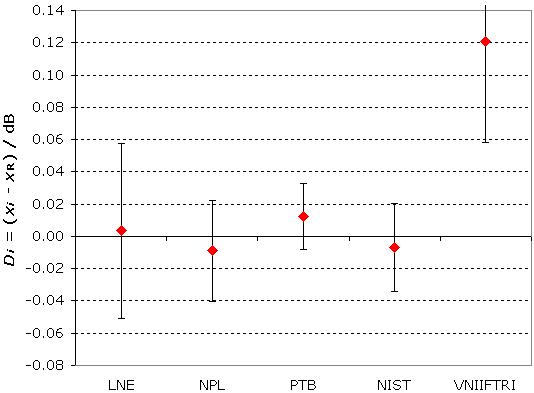Note that the terms Di are computed with the opposite sign in the Final Report.

MEASURAND : Excess noise ratio
NOISE SOURCE : TSA1
REFERENCE PLANE : R140
FREQUENCY : 13.5 GHz

Degrees of equivalence: Di and expanded uncertainty Ui (k = 2), both expressed in dB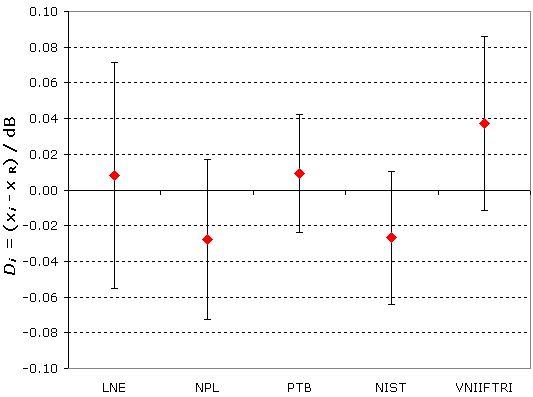Note that the terms Di are computed with the opposite sign in the Final Report.

MEASURAND : Excess noise ratio
NOISE SOURCE : TSA1
REFERENCE PLANE : R140
FREQUENCY : 15 GHz

Degrees of equivalence: Di and expanded uncertainty Ui (k = 2), both expressed in dB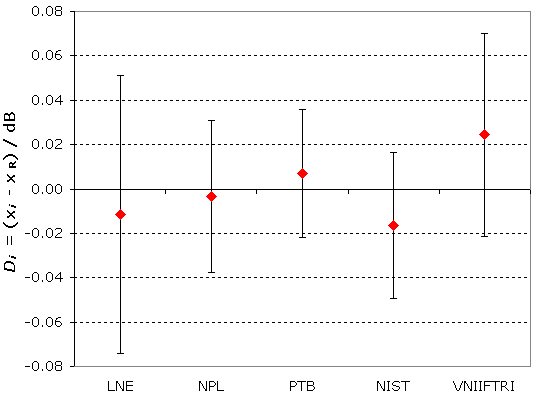Note that the terms Di are computed with the opposite sign in the Final Report.

MEASURAND : Excess noise ratio
NOISE SOURCE : TSA1
REFERENCE PLANE : R140
FREQUENCY : 17.5 GHz

Degrees of equivalence: Di and expanded uncertainty Ui (k = 2), both expressed in dB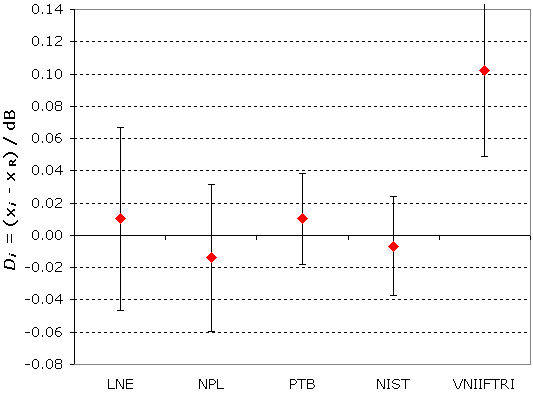Note that the terms Di are computed with the opposite sign in the Final Report.

MEASURAND : Excess noise ratio
NOISE SOURCE : TSA1
REFERENCE PLANE : R140
FREQUENCY : 18 GHz

Degrees of equivalence: Di and expanded uncertainty Ui (k = 2), both expressed in dB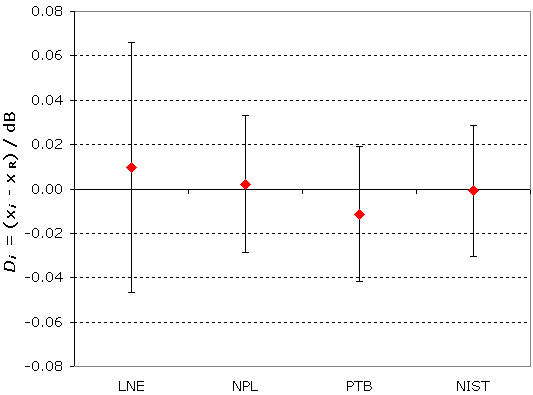Note that the terms Di are computed with the opposite sign in the Final Report.

MEASURAND : Excess noise ratio
NOISE SOURCE : TSB1
REFERENCE PLANE : PC-7 / R140
FREQUENCY : 12.4 GHz

Degrees of equivalence: Di and expanded uncertainty Ui (k = 2), both expressed in dB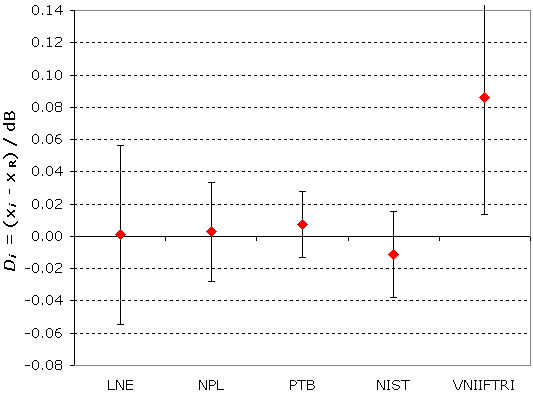Note that the terms Di are computed with the opposite sign in the Final Report.

MEASURAND : Excess noise ratio
NOISE SOURCE : TSB1
REFERENCE PLANE : PC-7 / R140
FREQUENCY : 13.5 GHz

Degrees of equivalence: Di and expanded uncertainty Ui (k = 2), both expressed in dB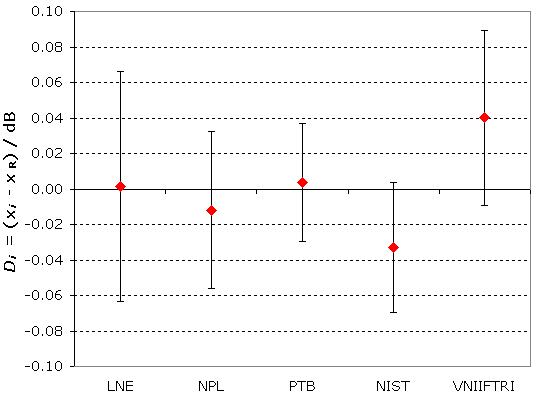Note that the terms Di are computed with the opposite sign in the Final Report.

MEASURAND : Excess noise ratio
NOISE SOURCE : TSB1
REFERENCE PLANE : PC-7 / R140
FREQUENCY : 15 GHz

Degrees of equivalence: Di and expanded uncertainty Ui (k = 2), both expressed in dB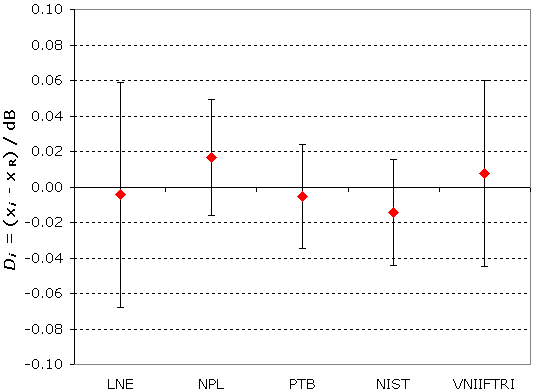Note that the terms Di are computed with the opposite sign in the Final Report.

MEASURAND : Excess noise ratio
NOISE SOURCE : TSB1
REFERENCE PLANE : PC-7 / R140
FREQUENCY : 17.5 GHz

Degrees of equivalence: Di and expanded uncertainty Ui (k = 2), both expressed in dB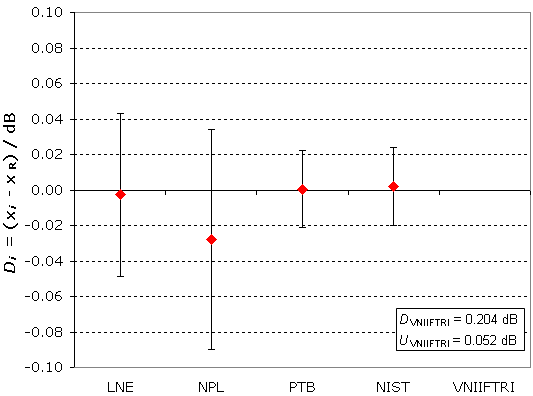Note that the terms Di are computed with the opposite sign in the Final Report.

MEASURAND : Excess noise ratio
NOISE SOURCE : TSB1
REFERENCE PLANE : PC-7 / R140
FREQUENCY : 18 GHz

Degrees of equivalence: Di and expanded uncertainty Ui (k = 2), both expressed in dB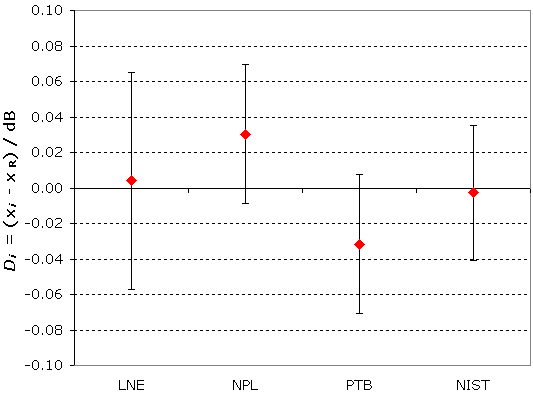Note that the terms Di are computed with the opposite sign in the Final Report.

MEASURAND : Excess noise ratio
NOISE SOURCE : TSB1
REFERENCE PLANE : PC-7
FREQUENCY : 12.4 GHz

Degrees of equivalence: Di and expanded uncertainty Ui (k = 2), both expressed in dB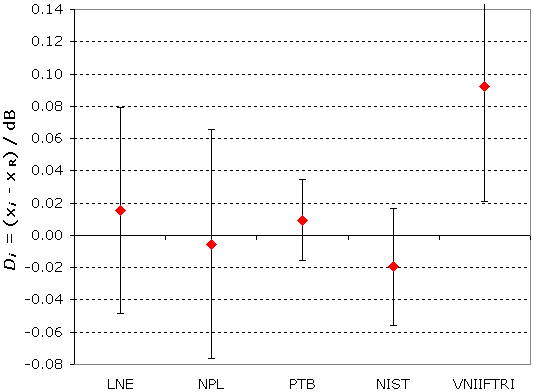Note that the terms Di are computed with the opposite sign in the Final Report.

MEASURAND : Excess noise ratio
NOISE SOURCE : TSB1
REFERENCE PLANE : PC-7
FREQUENCY : 13.5 GHz

Degrees of equivalence: Di and expanded uncertainty Ui (k = 2), both expressed in dB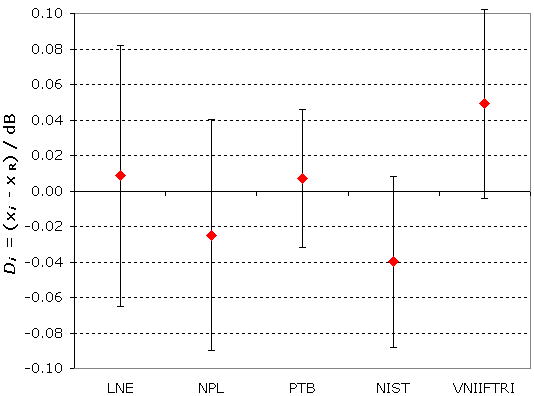Note that the terms Di are computed with the opposite sign in the Final Report.

MEASURAND : Excess noise ratio
NOISE SOURCE : TSB1
REFERENCE PLANE : PC-7
FREQUENCY : 15 GHz

Degrees of equivalence: Di and expanded uncertainty Ui (k = 2), both expressed in dB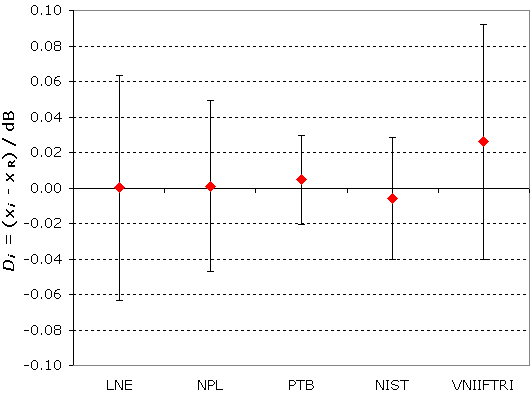Note that the terms Di are computed with the opposite sign in the Final Report.

MEASURAND : Excess noise ratio
NOISE SOURCE : TSB1
REFERENCE PLANE : PC-7
FREQUENCY : 17.5 GHz

Degrees of equivalence: Di and expanded uncertainty Ui (k = 2), both expressed in dB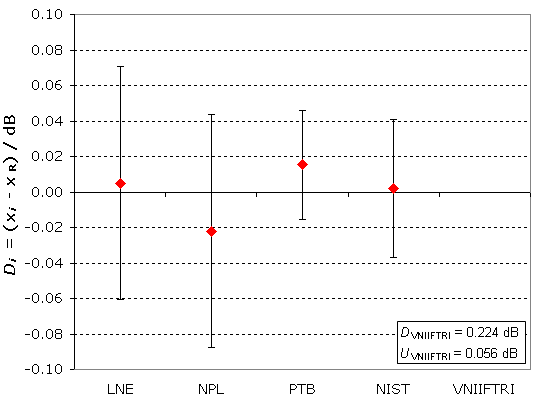Note that the terms Di are computed with the opposite sign in the Final Report.

MEASURAND : Excess noise ratio
NOISE SOURCE : TSB1
REFERENCE PLANE : PC-7
FREQUENCY : 18 GHz

Degrees of equivalence: Di and expanded uncertainty Ui (k = 2), both expressed in dBNote that the terms Di are computed with the opposite sign in the Final Report.

MEASURAND : Excess noise ratio
NOISE SOURCE : TSA2
REFERENCE PLANE : R140
FREQUENCY : 12.4 GHz

Degrees of equivalence: Di and expanded uncertainty Ui (k = 2), both expressed in dB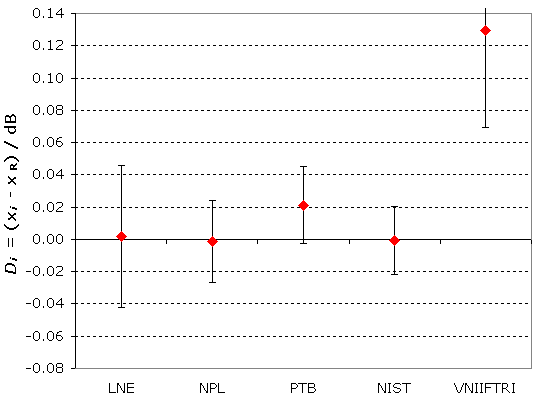Note that the terms Di are computed with the opposite sign in the Final Report.

MEASURAND : Excess noise ratio
NOISE SOURCE : TSA2
REFERENCE PLANE : R140
FREQUENCY : 13.5 GHz

Degrees of equivalence: Di and expanded uncertainty Ui (k = 2), both expressed in dB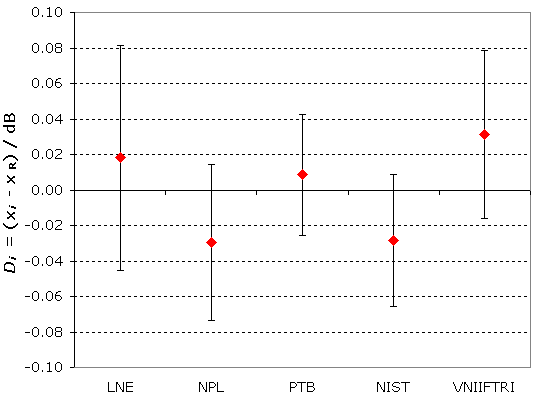Note that the terms Di are computed with the opposite sign in the Final Report.

MEASURAND : Excess noise ratio
NOISE SOURCE : TSA2
REFERENCE PLANE : R140
FREQUENCY : 15 GHz

Degrees of equivalence: Di and expanded uncertainty Ui (k = 2), both expressed in dB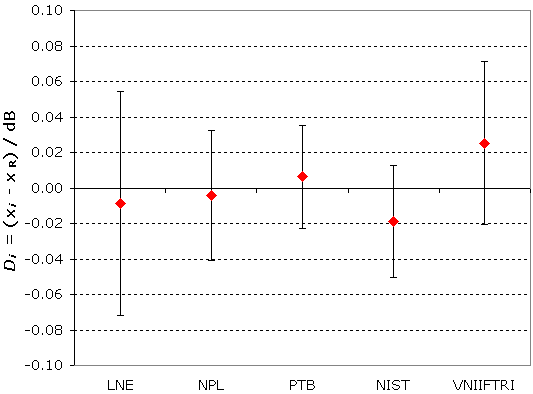Note that the terms Di are computed with the opposite sign in the Final Report.

MEASURAND : Excess noise ratio
NOISE SOURCE : TSA2
REFERENCE PLANE : R140
FREQUENCY : 17.5 GHz

Degrees of equivalence: Di and expanded uncertainty Ui (k = 2), both expressed in dB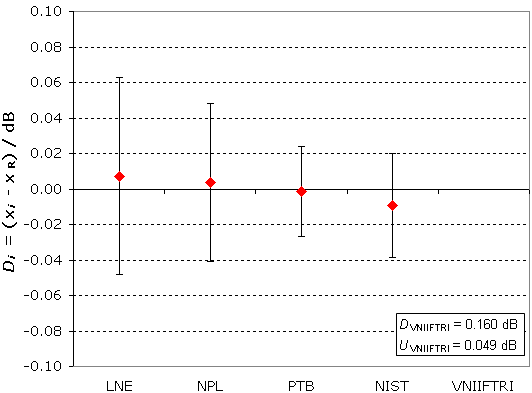Note that the terms Di are computed with the opposite sign in the Final Report.

MEASURAND : Excess noise ratio
NOISE SOURCE : TSA2
REFERENCE PLANE : R140
FREQUENCY : 18 GHz

Degrees of equivalence: Di and expanded uncertainty Ui (k = 2), both expressed in dB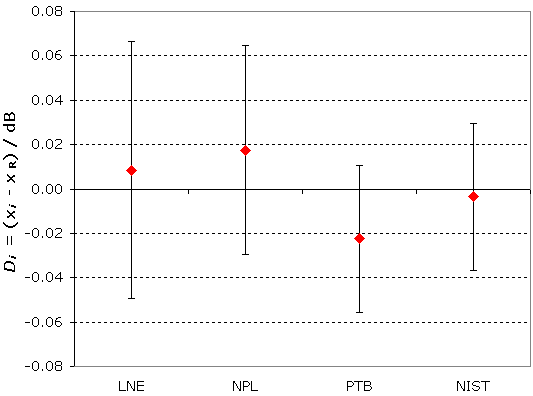Note that the terms Di are computed with the opposite sign in the Final Report.

MEASURAND : Excess noise ratio
NOISE SOURCE : TSB2
REFERENCE PLANE : PC-7 / R140
FREQUENCY : 12.4 GHz

Degrees of equivalence: Di and expanded uncertainty Ui (k = 2), both expressed in dB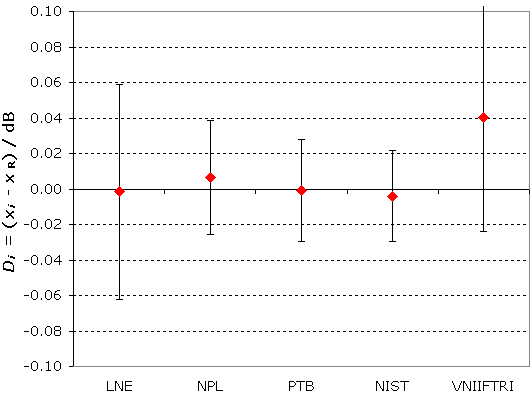Note that the terms Di are computed with the opposite sign in the Final Report.

MEASURAND : Excess noise ratio
NOISE SOURCE : TSB2
REFERENCE PLANE : PC-7 / R140
FREQUENCY : 13.5 GHz

Degrees of equivalence: Di and expanded uncertainty Ui (k = 2), both expressed in dB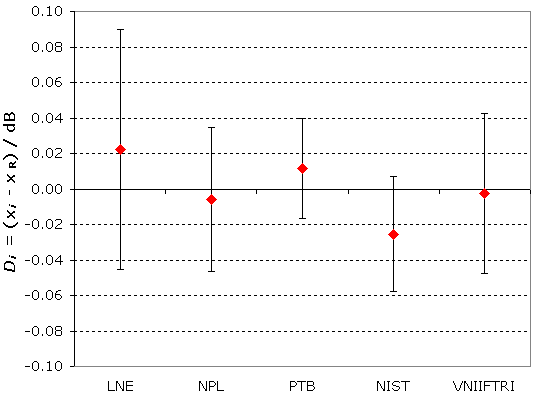Note that the terms Di are computed with the opposite sign in the Final Report.

MEASURAND : Excess noise ratio
NOISE SOURCE : TSB2
REFERENCE PLANE : PC-7 / R140
FREQUENCY : 15 GHz

Degrees of equivalence: Di and expanded uncertainty Ui (k = 2), both expressed in dB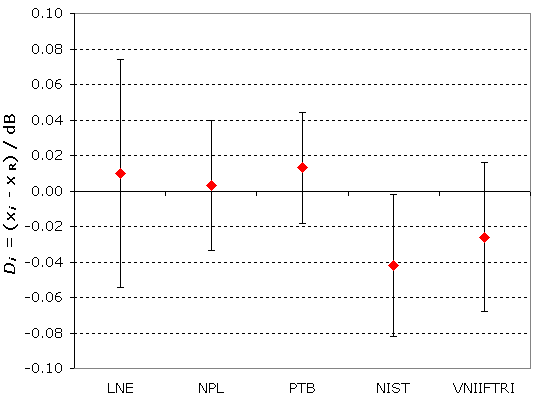Note that the terms Di are computed with the opposite sign in the Final Report.

MEASURAND : Excess noise ratio
NOISE SOURCE : TSB2
REFERENCE PLANE : PC-7 / R140
FREQUENCY : 17.5 GHz

Degrees of equivalence: Di and expanded uncertainty Ui (k = 2), both expressed in dB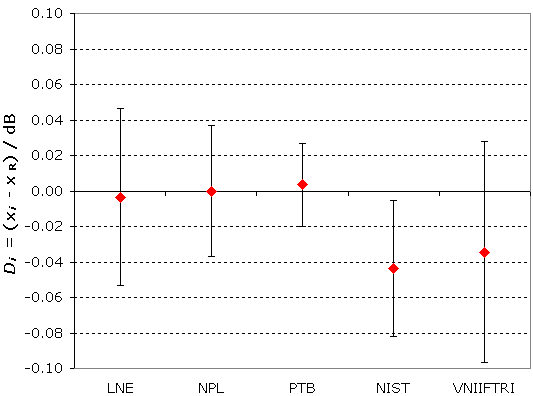Note that the terms Di are computed with the opposite sign in the Final Report.

MEASURAND : Excess noise ratio
NOISE SOURCE : TSB2
REFERENCE PLANE : PC-7 / R140
FREQUENCY : 18 GHz

Degrees of equivalence: Di and expanded uncertainty Ui (k = 2), both expressed in dB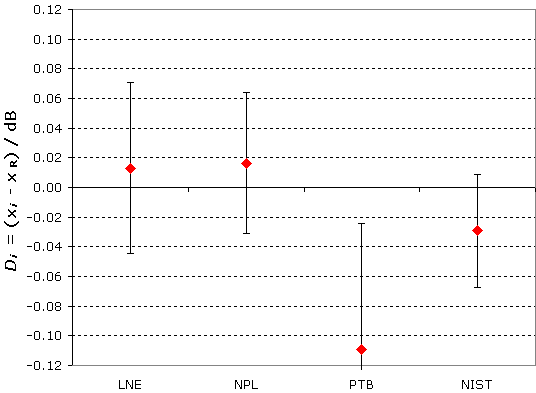Note that the terms Di are computed with the opposite sign in the Final Report.

MEASURAND : Excess noise ratio
NOISE SOURCE : TSB2
REFERENCE PLANE : PC-7
FREQUENCY : 12.4 GHz

Degrees of equivalence: Di and expanded uncertainty Ui (k = 2), both expressed in dB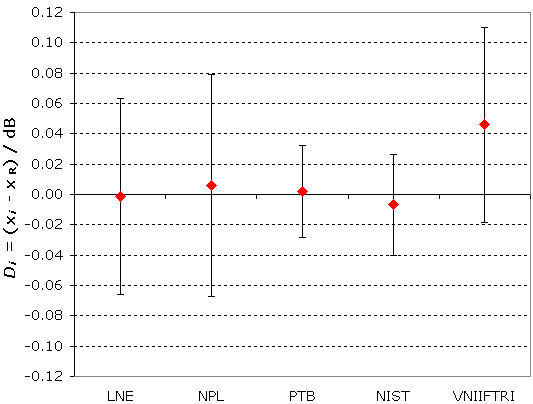Note that the terms Di are computed with the opposite sign in the Final Report.

MEASURAND : Excess noise ratio
NOISE SOURCE : TSB2
REFERENCE PLANE : PC-7
FREQUENCY : 13.5 GHz

Degrees of equivalence: Di and expanded uncertainty Ui (k = 2), both expressed in dB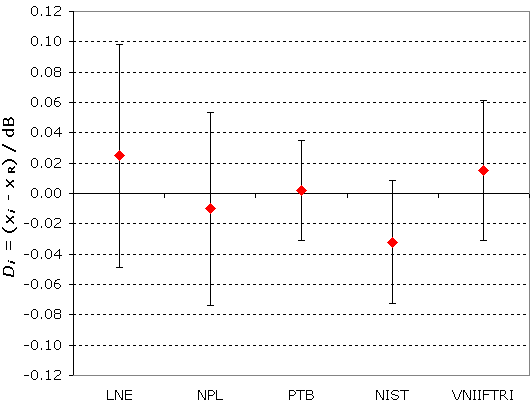Note that the terms Di are computed with the opposite sign in the Final Report.

MEASURAND : Excess noise ratio
NOISE SOURCE : TSB2
REFERENCE PLANE : PC-7
FREQUENCY : 15 GHz

Degrees of equivalence: Di and expanded uncertainty Ui (k = 2), both expressed in dB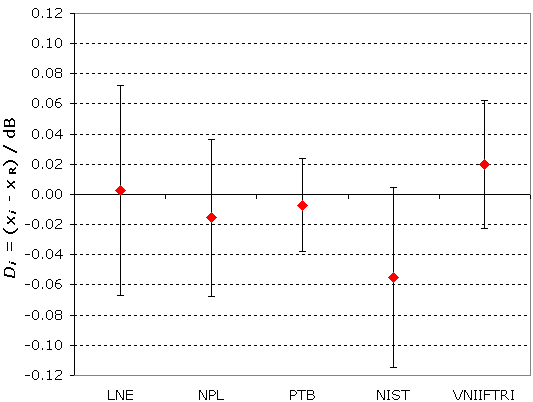Note that the terms Di are computed with the opposite sign in the Final Report.

MEASURAND : Excess noise ratio
NOISE SOURCE : TSB2
REFERENCE PLANE : PC-7
FREQUENCY : 17.5 GHz

Degrees of equivalence: Di and expanded uncertainty Ui (k = 2), both expressed in dB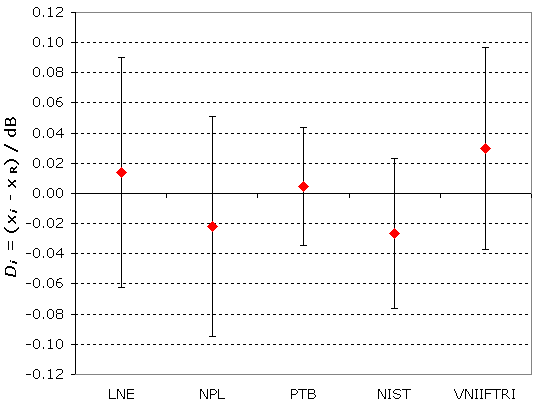Note that the terms Di are computed with the opposite sign in the Final Report.

MEASURAND : Excess noise ratio
NOISE SOURCE : TSB2
REFERENCE PLANE : PC-7
FREQUENCY : 18 GHz

Degrees of equivalence: Di and expanded uncertainty Ui (k = 2), both expressed in dB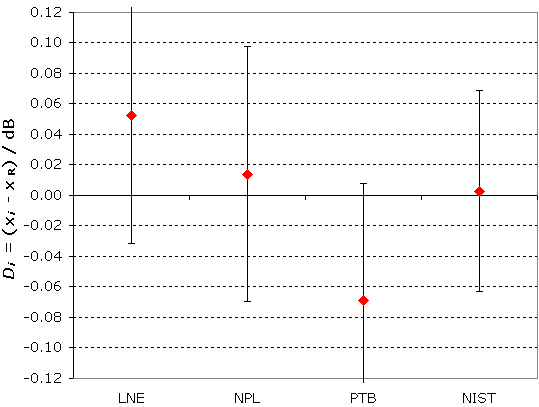Note that the terms Di are computed with the opposite sign in the Final Report.

Comparison
Comparison close
Results

MEASURAND : Excess noise ratio
NOISE SOURCE : TSA1
REFERENCE PLANE : R140
FREQUENCY : 12.4 GHz

 Lab i Di Ui / dB / dB LNE 0.004 0.054 NPL -0.009 0.031 PTB 0.012 0.020 NIST -0.007 0.027 VNIIFTRI 0.121 0.063

Results are presented under A4 printable format in Summary Results (.PDF file).

MEASURAND : Excess noise ratio
NOISE SOURCE : TSA1
REFERENCE PLANE : R140
FREQUENCY : 13.5 GHz

 Lab i Di Ui / dB / dB LNE 0.008 0.063 NPL -0.028 0.045 PTB 0.009 0.033 NIST -0.027 0.037 VNIIFTRI 0.037 0.049

Results are presented under A4 printable format in Summary Results (.PDF file).

MEASURAND : Excess noise ratio
NOISE SOURCE : TSA1
REFERENCE PLANE : R140
FREQUENCY : 15 GHz

 Lab i Di Ui / dB / dB LNE -0.012 0.063 NPL -0.004 0.034 PTB 0.007 0.029 NIST -0.017 0.033 VNIIFTRI 0.024 0.046

Results are presented under A4 printable format in Summary Results (.PDF file).

MEASURAND : Excess noise ratio
NOISE SOURCE : TSA1
REFERENCE PLANE : R140
FREQUENCY : 17.5 GHz

 Lab i Di Ui / dB / dB LNE 0.010 0.056 NPL -0.014 0.045 PTB 0.010 0.028 NIST -0.007 0.031 VNIIFTRI 0.102 0.053

Results are presented under A4 printable format in Summary Results (.PDF file).

MEASURAND : Excess noise ratio
NOISE SOURCE : TSA1
REFERENCE PLANE : R140
FREQUENCY : 18 GHz

 Lab i Di Ui / dB / dB LNE 0.010 0.056 NPL 0.002 0.031 PTB -0.011 0.030 NIST -0.001 0.030

Results are presented under A4 printable format in Summary Results (.PDF file).

MEASURAND : Excess noise ratio
NOISE SOURCE : TSB1
REFERENCE PLANE : PC-7 / R140
FREQUENCY : 12.4 GHz

 Lab i Di Ui / dB / dB LNE 0.001 0.055 NPL 0.003 0.031 PTB 0.007 0.021 NIST -0.011 0.027 VNIIFTRI 0.086 0.072

Results are presented under A4 printable format in Summary Results (.PDF file).

MEASURAND : Excess noise ratio
NOISE SOURCE : TSB1
REFERENCE PLANE : PC-7 / R140
FREQUENCY : 13.5 GHz

 Lab i Di Ui / dB / dB LNE 0.001 0.065 NPL -0.012 0.044 PTB 0.004 0.033 NIST -0.033 0.037 VNIIFTRI 0.040 0.050

Results are presented under A4 printable format in Summary Results (.PDF file).

MEASURAND : Excess noise ratio
NOISE SOURCE : TSB1
REFERENCE PLANE : PC-7 / R140
FREQUENCY : 15 GHz

 Lab i Di Ui / dB / dB LNE -0.004 0.063 NPL 0.017 0.033 PTB -0.005 0.029 NIST -0.014 0.030 VNIIFTRI 0.008 0.052

Results are presented under A4 printable format in Summary Results (.PDF file).

MEASURAND : Excess noise ratio
NOISE SOURCE : TSB1
REFERENCE PLANE : PC-7 / R140
FREQUENCY : 17.5 GHz

 Lab i Di Ui / dB / dB LNE -0.003 0.046 NPL -0.028 0.062 PTB 0.001 0.022 NIST 0.002 0.022 VNIIFTRI 0.204 0.052

Results are presented under A4 printable format in Summary Results (.PDF file).

MEASURAND : Excess noise ratio
NOISE SOURCE : TSB1
REFERENCE PLANE : PC-7 / R140
FREQUENCY : 18 GHz

 Lab i Di Ui / dB / dB LNE 0.004 0.061 NPL 0.030 0.039 PTB -0.032 0.039 NIST -0.003 0.038

Results are presented under A4 printable format in Summary Results (.PDF file).

MEASURAND : Excess noise ratio
NOISE SOURCE : TSB1
REFERENCE PLANE : PC-7
FREQUENCY : 12.4 GHz

 Lab i Di Ui / dB / dB LNE 0.016 0.064 NPL -0.005 0.071 PTB 0.010 0.025 NIST -0.019 0.036 VNIIFTRI 0.093 0.072

Results are presented under A4 printable format in Summary Results (.PDF file).

MEASURAND : Excess noise ratio
NOISE SOURCE : TSB1
REFERENCE PLANE : PC-7
FREQUENCY : 13.5 GHz

 Lab i Di Ui / dB / dB LNE 0.008 0.073 NPL -0.025 0.065 PTB 0.007 0.039 NIST -0.040 0.048 VNIIFTRI 0.049 0.053

Results are presented under A4 printable format in Summary Results (.PDF file).

MEASURAND : Excess noise ratio
NOISE SOURCE : TSB1
REFERENCE PLANE : PC-7
FREQUENCY : 15 GHz

 Lab i Di Ui / dB / dB LNE 0.000 0.063 NPL 0.001 0.048 PTB 0.005 0.025 NIST -0.006 0.034 VNIIFTRI 0.026 0.066

Results are presented under A4 printable format in Summary Results (.PDF file).

MEASURAND : Excess noise ratio
NOISE SOURCE : TSB1
REFERENCE PLANE : PC-7
FREQUENCY : 17.5 GHz

 Lab i Di Ui / dB / dB LNE 0.005 0.066 NPL -0.022 0.066 PTB 0.015 0.031 NIST 0.002 0.039 VNIIFTRI 0.224 0.056

Results are presented under A4 printable format in Summary Results (.PDF file).

MEASURAND : Excess noise ratio
NOISE SOURCE : TSB1
REFERENCE PLANE : PC-7
FREQUENCY : 18 GHz

 Lab i Di Ui / dB / dB LNE 0.013 0.071 NPL 0.038 0.065 PTB -0.039 0.044 NIST -0.011 0.048

Results are presented under A4 printable format in Summary Results (.PDF file).

MEASURAND : Excess noise ratio
NOISE SOURCE : TSA2
REFERENCE PLANE : R140
FREQUENCY : 12.4 GHz

 Lab i Di Ui / dB / dB LNE 0.002 0.044 NPL -0.001 0.025 PTB 0.021 0.024 NIST -0.001 0.021 VNIIFTRI 0.129 0.060

Results are presented under A4 printable format in Summary Results (.PDF file).

MEASURAND : Excess noise ratio
NOISE SOURCE : TSA2
REFERENCE PLANE : R140
FREQUENCY : 13.5 GHz

 Lab i Di Ui / dB / dB LNE 0.018 0.063 NPL -0.030 0.044 PTB 0.008 0.034 NIST -0.029 0.037 VNIIFTRI 0.031 0.047

Results are presented under A4 printable format in Summary Results (.PDF file).

MEASURAND : Excess noise ratio
NOISE SOURCE : TSA2
REFERENCE PLANE : R140
FREQUENCY : 15 GHz

 Lab i Di Ui / dB / dB LNE -0.009 0.063 NPL -0.004 0.037 PTB 0.006 0.029 NIST -0.019 0.032 VNIIFTRI 0.025 0.046

Results are presented under A4 printable format in Summary Results (.PDF file).

MEASURAND : Excess noise ratio
NOISE SOURCE : TSA2
REFERENCE PLANE : R140
FREQUENCY : 17.5 GHz

 Lab i Di Ui / dB / dB LNE 0.007 0.056 NPL 0.004 0.044 PTB -0.001 0.025 NIST -0.009 0.029 VNIIFTRI 0.160 0.049

Results are presented under A4 printable format in Summary Results (.PDF file).

MEASURAND : Excess noise ratio
NOISE SOURCE : TSA2
REFERENCE PLANE : R140
FREQUENCY : 18 GHz

 Lab i Di Ui / dB / dB LNE 0.009 0.058 NPL 0.018 0.047 PTB -0.022 0.033 NIST -0.003 0.033

Results are presented under A4 printable format in Summary Results (.PDF file).

MEASURAND : Excess noise ratio
NOISE SOURCE : TSB2
REFERENCE PLANE : PC-7 / R140
FREQUENCY : 12.4 GHz

 Lab i Di Ui / dB / dB LNE -0.002 0.061 NPL 0.007 0.032 PTB -0.001 0.029 NIST -0.004 0.026 VNIIFTRI 0.040 0.064

Results are presented under A4 printable format in Summary Results (.PDF file).

MEASURAND : Excess noise ratio
NOISE SOURCE : TSB2
REFERENCE PLANE : PC-7 / R140
FREQUENCY : 13.5 GHz

 Lab i Di Ui / dB / dB LNE 0.023 0.068 NPL -0.006 0.041 PTB 0.011 0.028 NIST -0.026 0.032 VNIIFTRI -0.003 0.045

Results are presented under A4 printable format in Summary Results (.PDF file).

MEASURAND : Excess noise ratio
NOISE SOURCE : TSB2
REFERENCE PLANE : PC-7 / R140
FREQUENCY : 15 GHz

 Lab i Di Ui / dB / dB LNE 0.010 0.064 NPL 0.003 0.036 PTB 0.013 0.031 NIST -0.042 0.040 VNIIFTRI -0.026 0.042

Results are presented under A4 printable format in Summary Results (.PDF file).

MEASURAND : Excess noise ratio
NOISE SOURCE : TSB2
REFERENCE PLANE : PC-7 / R140
FREQUENCY : 17.5 GHz

 Lab i Di Ui / dB / dB LNE -0.003 0.050 NPL 0.000 0.037 PTB 0.003 0.023 NIST -0.043 0.038 VNIIFTRI -0.035 0.062

Results are presented under A4 printable format in Summary Results (.PDF file).

MEASURAND : Excess noise ratio
NOISE SOURCE : TSB2
REFERENCE PLANE : PC-7 / R140
FREQUENCY : 18 GHz

 Lab i Di Ui / dB / dB LNE 0.013 0.058 NPL 0.016 0.048 PTB -0.109 0.085 NIST -0.029 0.038

Results are presented under A4 printable format in Summary Results (.PDF file).

MEASURAND : Excess noise ratio
NOISE SOURCE : TSB2
REFERENCE PLANE : PC-7
FREQUENCY : 12.4 GHz

 Lab i Di Ui / dB / dB LNE -0.001 0.065 NPL 0.006 0.073 PTB 0.002 0.030 NIST -0.007 0.033 VNIIFTRI 0.046 0.064

Results are presented under A4 printable format in Summary Results (.PDF file).

MEASURAND : Excess noise ratio
NOISE SOURCE : TSB2
REFERENCE PLANE : PC-7
FREQUENCY : 13.5 GHz

 Lab i Di Ui / dB / dB LNE 0.025 0.073 NPL -0.010 0.064 PTB 0.002 0.033 NIST -0.032 0.041 VNIIFTRI 0.015 0.046

Results are presented under A4 printable format in Summary Results (.PDF file).

MEASURAND : Excess noise ratio
NOISE SOURCE : TSB2
REFERENCE PLANE : PC-7
FREQUENCY : 15 GHz

 Lab i Di Ui / dB / dB LNE 0.003 0.070 NPL -0.016 0.052 PTB -0.007 0.031 NIST -0.055 0.060 VNIIFTRI 0.020 0.042

Results are presented under A4 printable format in Summary Results (.PDF file).

MEASURAND : Excess noise ratio
NOISE SOURCE : TSB2
REFERENCE PLANE : PC-7
FREQUENCY : 17.5 GHz

 Lab i Di Ui / dB / dB LNE 0.014 0.076 NPL -0.022 0.073 PTB 0.005 0.039 NIST -0.026 0.050 VNIIFTRI 0.030 0.067

Results are presented under A4 printable format in Summary Results (.PDF file).

MEASURAND : Excess noise ratio
NOISE SOURCE : TSB2
REFERENCE PLANE : PC-7
FREQUENCY : 18 GHz

 Lab i Di Ui / dB / dB LNE 0.052 0.084 NPL 0.014 0.084 PTB -0.069 0.077 NIST 0.003 0.066

Results are presented under A4 printable format in Summary Results (.PDF file).

Comparison
Comparison close
CC comparison
 Metrology area, Sub-field Electricity and Magnetism, Radio frequencies Description Excess noise ratio / noise temperature Time of measurements 1998 - 2000 Status Approved for equivalence
 Final Reports of the comparisons Measurand Excess noise ratio Parameters Frequency: 12.4 GHz, 13.5 GHz, 15 GHz, 17.5 GHz and 18 GHz Transfer device Different noise sources
 Comparison type Key Comparison Consultative Committee CCEM (Consultative Committee for Electricity and Magnetism) Conducted by CCEM (Consultative Committee for Electricity and Magnetism) Comments A subsequent bilateral comparison was decided between VNIIFTRI and PTB. It is registered into the BIPM key comparison database under the identifier CCEM.RF-K9.1.   Results published on 13 February 2006 Excess noise ratio Pilot institute LNE Laboratoire national de métrologie et d'essais France Contact person D. Allal +33 (0) 1 30 69 21 50
First Name Last Name
wwww@ww.www +356719836 Institute 1 Institute 1 Khmelnitskiy
Pilot laboratory
LNE

Laboratoire national de métrologie et d'essais, France, EURAMET

 NIST National Institute of Standards and Technology, United States, SIM
 NPL National Physical Laboratory, United Kingdom, EURAMET
 PTB Physikalisch-Technische Bundesanstalt, Germany, EURAMET
 VNIIFTRI Institute of Physical Technical and Radiotechnical Measurements, Rosstandart, Russian Federation, COOMET
Comparison
Comparison close
Results

MEASURAND : Excess noise ratio
NOISE SOURCE : TSA1
REFERENCE PLANE : R140
FREQUENCY : 12.4 GHz

 The key comparison reference value xR is calculated as the unweighted mean of the participants results with exclusion of outliers. Its standard uncertainty uR is computed as given in page 112 of the Final Report. xR = 15.696 dB, uR = 0.005 dB

 The degree of equivalence of laboratory i with respect to the key comparison reference value is given by two terms both expressed in dB: Di = (xi - xR) and its expanded uncertainty Ui (k = 2). The equations for the computation of Ui are given in page 112 of the Final Report.

 The degree of equivalence between two laboratories i and j is given by two terms both expressed in dB:Dij = (Di - Dj) and its expanded uncertainty Uij (k = 2) with Uij = 2(ui2 + uj2)1/2.

Note that the terms Di and Dij are computed with the opposite sign in the Final Report.

MEASURAND : Excess noise ratio
NOISE SOURCE : TSA1
REFERENCE PLANE : R140
FREQUENCY : 13.5 GHz

 The key comparison reference value xR is calculated as the unweighted mean of the participants results with exclusion of outliers. Its standard uncertainty uR is computed as given in page 112 of the Final Report. xR = 15.710 dB, uR = 0.012 dB

 The degree of equivalence of laboratory i with respect to the key comparison reference value is given by two terms both expressed in dB: Di = (xi - xR) and its expanded uncertainty Ui (k = 2). The equations for the computation of Ui are given in page 112 of the Final Report.

 The degree of equivalence between two laboratories i and j is given by two terms both expressed in dB:Dij = (Di - Dj) and its expanded uncertainty Uij (k = 2) with Uij = 2(ui2 + uj2)1/2.

Note that the terms Di and Dij are computed with the opposite sign in the Final Report.

MEASURAND : Excess noise ratio
NOISE SOURCE : TSA1
REFERENCE PLANE : R140
FREQUENCY : 15 GHz

 The key comparison reference value xR is calculated as the unweighted mean of the participants results with exclusion of outliers. Its standard uncertainty uR is computed as given in page 112 of the Final Report. xR = 15.693 dB, uR = 0.007 dB

 The degree of equivalence of laboratory i with respect to the key comparison reference value is given by two terms both expressed in dB: Di = (xi - xR) and its expanded uncertainty Ui (k = 2). The equations for the computation of Ui are given in page 112 of the Final Report.

 The degree of equivalence between two laboratories i and j is given by two terms both expressed in dB:Dij = (Di - Dj) and its expanded uncertainty Uij (k = 2) with Uij = 2(ui2 + uj2)1/2.

Note that the terms Di and Dij are computed with the opposite sign in the Final Report.

MEASURAND : Excess noise ratio
NOISE SOURCE : TSA1
REFERENCE PLANE : R140
FREQUENCY : 17.5 GHz

 The key comparison reference value xR is calculated as the unweighted mean of the participants results with exclusion of outliers. Its standard uncertainty uR is computed as given in page 112 of the Final Report. xR = 15.673 dB, uR = 0.006 dB

 The degree of equivalence of laboratory i with respect to the key comparison reference value is given by two terms both expressed in dB: Di = (xi - xR) and its expanded uncertainty Ui (k = 2). The equations for the computation of Ui are given in page 112 of the Final Report.

 The degree of equivalence between two laboratories i and j is given by two terms both expressed in dB:Dij = (Di - Dj) and its expanded uncertainty Uij (k = 2) with Uij = 2(ui2 + uj2)1/2.

Note that the terms Di and Dij are computed with the opposite sign in the Final Report.

MEASURAND : Excess noise ratio
NOISE SOURCE : TSA1
REFERENCE PLANE : R140
FREQUENCY : 18 GHz

 The key comparison reference value xR is calculated as the unweighted mean of the participants results with exclusion of outliers. Its standard uncertainty uR is computed as given in page 112 of the Final Report. xR = 15.656 dB, uR = 0.004 dB

 The degree of equivalence of laboratory i with respect to the key comparison reference value is given by two terms both expressed in dB: Di = (xi - xR) and its expanded uncertainty Ui (k = 2). The equations for the computation of Ui are given in page 112 of the Final Report.

 The degree of equivalence between two laboratories i and j is given by two terms both expressed in dB:Dij = (Di - Dj) and its expanded uncertainty Uij (k = 2) with Uij = 2(ui2 + uj2)1/2.

Note that the terms Di and Dij are computed with the opposite sign in the Final Report.

MEASURAND : Excess noise ratio
NOISE SOURCE : TSB1
REFERENCE PLANE : PC-7 / R140
FREQUENCY : 12.4 GHz

 The key comparison reference value xR is calculated as the unweighted mean of the participants results with exclusion of outliers. Its standard uncertainty uR is computed as given in page 112 of the Final Report. xR = 15.784 dB, uR = 0.004 dB

 The degree of equivalence of laboratory i with respect to the key comparison reference value is given by two terms both expressed in dB: Di = (xi - xR) and its expanded uncertainty Ui (k = 2). The equations for the computation of Ui are given in page 112 of the Final Report.

 The degree of equivalence between two laboratories i and j is given by two terms both expressed in dB:Dij = (Di - Dj) and its expanded uncertainty Uij (k = 2) with Uij = 2(ui2 + uj2)1/2.

Note that the terms Di and Dij are computed with the opposite sign in the Final Report.

MEASURAND : Excess noise ratio
NOISE SOURCE : TSB1
REFERENCE PLANE : PC-7 / R140
FREQUENCY : 13.5 GHz

 The key comparison reference value xR is calculated as the unweighted mean of the participants results with exclusion of outliers. Its standard uncertainty uR is computed as given in page 112 of the Final Report. xR = 15.834 dB, uR = 0.012 dB

 The degree of equivalence of laboratory i with respect to the key comparison reference value is given by two terms both expressed in dB: Di = (xi - xR) and its expanded uncertainty Ui (k = 2). The equations for the computation of Ui are given in page 112 of the Final Report.

 The degree of equivalence between two laboratories i and j is given by two terms both expressed in dB:Dij = (Di - Dj) and its expanded uncertainty Uij (k = 2) with Uij = 2(ui2 + uj2)1/2.

Note that the terms Di and Dij are computed with the opposite sign in the Final Report.

MEASURAND : Excess noise ratio
NOISE SOURCE : TSB1
REFERENCE PLANE : PC-7 / R140
FREQUENCY : 15 GHz

 The key comparison reference value xR is calculated as the unweighted mean of the participants results with exclusion of outliers. Its standard uncertainty uR is computed as given in page 112 of the Final Report. xR = 15.840 dB, uR = 0.005 dB

 The degree of equivalence of laboratory i with respect to the key comparison reference value is given by two terms both expressed in dB: Di = (xi - xR) and its expanded uncertainty Ui (k = 2). The equations for the computation of Ui are given in page 112 of the Final Report.

 The degree of equivalence between two laboratories i and j is given by two terms both expressed in dB:Dij = (Di - Dj) and its expanded uncertainty Uij (k = 2) with Uij = 2(ui2 + uj2)1/2.

Note that the terms Di and Dij are computed with the opposite sign in the Final Report.

MEASURAND : Excess noise ratio
NOISE SOURCE : TSB1
REFERENCE PLANE : PC-7 / R140
FREQUENCY : 17.5 GHz

 The key comparison reference value xR is calculated as the unweighted mean of the participants results with exclusion of outliers. Its standard uncertainty uR is computed as given in page 112 of the Final Report. xR = 15.695 dB, uR = 0.001 dB

 The degree of equivalence of laboratory i with respect to the key comparison reference value is given by two terms both expressed in dB: Di = (xi - xR) and its expanded uncertainty Ui (k = 2). The equations for the computation of Ui are given in page 112 of the Final Report.

 The degree of equivalence between two laboratories i and j is given by two terms both expressed in dB:Dij = (Di - Dj) and its expanded uncertainty Uij (k = 2) with Uij = 2(ui2 + uj2)1/2.

Note that the terms Di and Dij are computed with the opposite sign in the Final Report.

MEASURAND : Excess noise ratio
NOISE SOURCE : TSB1
REFERENCE PLANE : PC-7 / R140
FREQUENCY : 18 GHz

 The key comparison reference value xR is calculated as the unweighted mean of the participants results with exclusion of outliers. Its standard uncertainty uR is computed as given in page 112 of the Final Report. xR = 15.578 dB, uR = 0.013 dB

 The degree of equivalence of laboratory i with respect to the key comparison reference value is given by two terms both expressed in dB: Di = (xi - xR) and its expanded uncertainty Ui (k = 2). The equations for the computation of Ui are given in page 112 of the Final Report.

 The degree of equivalence between two laboratories i and j is given by two terms both expressed in dB:Dij = (Di - Dj) and its expanded uncertainty Uij (k = 2) with Uij = 2(ui2 + uj2)1/2.

Note that the terms Di and Dij are computed with the opposite sign in the Final Report.

MEASURAND : Excess noise ratio
NOISE SOURCE : TSB1
REFERENCE PLANE : PC-7
FREQUENCY : 12.4 GHz

 The key comparison reference value xR is calculated as the unweighted mean of the participants results with exclusion of outliers. Its standard uncertainty uR is computed as given in page 112 of the Final Report. xR = 15.862 dB, uR = 0.008 dB

 The degree of equivalence of laboratory i with respect to the key comparison reference value is given by two terms both expressed in dB: Di = (xi - xR) and its expanded uncertainty Ui (k = 2). The equations for the computation of Ui are given in page 112 of the Final Report.

 The degree of equivalence between two laboratories i and j is given by two terms both expressed in dB:Dij = (Di - Dj) and its expanded uncertainty Uij (k = 2) with Uij = 2(ui2 + uj2)1/2.

Note that the terms Di and Dij are computed with the opposite sign in the Final Report.

MEASURAND : Excess noise ratio
NOISE SOURCE : TSB1
REFERENCE PLANE : PC-7
FREQUENCY : 13.5 GHz

 The key comparison reference value xR is calculated as the unweighted mean of the participants results with exclusion of outliers. Its standard uncertainty uR is computed as given in page 112 of the Final Report. xR = 15.921 dB, uR = 0.015 dB

 The degree of equivalence of laboratory i with respect to the key comparison reference value is given by two terms both expressed in dB: Di = (xi - xR) and its expanded uncertainty Ui (k = 2). The equations for the computation of Ui are given in page 112 of the Final Report.

 The degree of equivalence between two laboratories i and j is given by two terms both expressed in dB:Dij = (Di - Dj) and its expanded uncertainty Uij (k = 2) with Uij = 2(ui2 + uj2)1/2.

Note that the terms Di and Dij are computed with the opposite sign in the Final Report.

MEASURAND : Excess noise ratio
NOISE SOURCE : TSB1
REFERENCE PLANE : PC-7
FREQUENCY : 15 GHz

 The key comparison reference value xR is calculated as the unweighted mean of the participants results with exclusion of outliers. Its standard uncertainty uR is computed as given in page 112 of the Final Report. xR = 15.956 dB, uR = 0.002 dB

 The degree of equivalence of laboratory i with respect to the key comparison reference value is given by two terms both expressed in dB: Di = (xi - xR) and its expanded uncertainty Ui (k = 2). The equations for the computation of Ui are given in page 112 of the Final Report.

 The degree of equivalence between two laboratories i and j is given by two terms both expressed in dB:Dij = (Di - Dj) and its expanded uncertainty Uij (k = 2) with Uij = 2(ui2 + uj2)1/2.

Note that the terms Di and Dij are computed with the opposite sign in the Final Report.

MEASURAND : Excess noise ratio
NOISE SOURCE : TSB1
REFERENCE PLANE : PC-7
FREQUENCY : 17.5 GHz

 The key comparison reference value xR is calculated as the unweighted mean of the participants results with exclusion of outliers. Its standard uncertainty uR is computed as given in page 112 of the Final Report. xR = 15.831 dB, uR = 0.008 dB

 The degree of equivalence of laboratory i with respect to the key comparison reference value is given by two terms both expressed in dB: Di = (xi - xR) and its expanded uncertainty Ui (k = 2). The equations for the computation of Ui are given in page 112 of the Final Report.

 The degree of equivalence between two laboratories i and j is given by two terms both expressed in dB:Dij = (Di - Dj) and its expanded uncertainty Uij (k = 2) with Uij = 2(ui2 + uj2)1/2.

Note that the terms Di and Dij are computed with the opposite sign in the Final Report.

MEASURAND : Excess noise ratio
NOISE SOURCE : TSB1
REFERENCE PLANE : PC-7
FREQUENCY : 18 GHz

 The key comparison reference value xR is calculated as the unweighted mean of the participants results with exclusion of outliers. Its standard uncertainty uR is computed as given in page 112 of the Final Report. xR = 15.728 dB, uR = 0.016 dB

 The degree of equivalence of laboratory i with respect to the key comparison reference value is given by two terms both expressed in dB: Di = (xi - xR) and its expanded uncertainty Ui (k = 2). The equations for the computation of Ui are given in page 112 of the Final Report.

 The degree of equivalence between two laboratories i and j is given by two terms both expressed in dB:Dij = (Di - Dj) and its expanded uncertainty Uij (k = 2) with Uij = 2(ui2 + uj2)1/2.

Note that the terms Di and Dij are computed with the opposite sign in the Final Report.

MEASURAND : Excess noise ratio
NOISE SOURCE : TSA2
REFERENCE PLANE : R140
FREQUENCY : 12.4 GHz

 The key comparison reference value xR is calculated as the unweighted mean of the participants results with exclusion of outliers. Its standard uncertainty uR is computed as given in page 112 of the Final Report. xR = 15.759 dB, uR = 0.001 dB

 The degree of equivalence of laboratory i with respect to the key comparison reference value is given by two terms both expressed in dB: Di = (xi - xR) and its expanded uncertainty Ui (k = 2). The equations for the computation of Ui are given in page 112 of the Final Report.

 The degree of equivalence between two laboratories i and j is given by two terms both expressed in dB:Dij = (Di - Dj) and its expanded uncertainty Uij (k = 2) with Uij = 2(ui2 + uj2)1/2.

Note that the terms Di and Dij are computed with the opposite sign in the Final Report.

MEASURAND : Excess noise ratio
NOISE SOURCE : TSA2
REFERENCE PLANE : R140
FREQUENCY : 13.5 GHz

 The key comparison reference value xR is calculated as the unweighted mean of the participants results with exclusion of outliers. Its standard uncertainty uR is computed as given in page 112 of the Final Report. xR = 15.785 dB, uR = 0.012 dB

 The degree of equivalence of laboratory i with respect to the key comparison reference value is given by two terms both expressed in dB: Di = (xi - xR) and its expanded uncertainty Ui (k = 2). The equations for the computation of Ui are given in page 112 of the Final Report.

 The degree of equivalence between two laboratories i and j is given by two terms both expressed in dB:Dij = (Di - Dj) and its expanded uncertainty Uij (k = 2) with Uij = 2(ui2 + uj2)1/2.

Note that the terms Di and Dij are computed with the opposite sign in the Final Report.

MEASURAND : Excess noise ratio
NOISE SOURCE : TSA2
REFERENCE PLANE : R140
FREQUENCY : 15 GHz

 The key comparison reference value xR is calculated as the unweighted mean of the participants results with exclusion of outliers. Its standard uncertainty uR is computed as given in page 112 of the Final Report. xR = 15.770 dB, uR = 0.007 dB

 The degree of equivalence of laboratory i with respect to the key comparison reference value is given by two terms both expressed in dB: Di = (xi - xR) and its expanded uncertainty Ui (k = 2). The equations for the computation of Ui are given in page 112 of the Final Report.

 The degree of equivalence between two laboratories i and j is given by two terms both expressed in dB:Dij = (Di - Dj) and its expanded uncertainty Uij (k = 2) with Uij = 2(ui2 + uj2)1/2.

Note that the terms Di and Dij are computed with the opposite sign in the Final Report.

MEASURAND : Excess noise ratio
NOISE SOURCE : TSA2
REFERENCE PLANE : R140
FREQUENCY : 17.5 GHz

 The key comparison reference value xR is calculated as the unweighted mean of the participants results with exclusion of outliers. Its standard uncertainty uR is computed as given in page 112 of the Final Report. xR = 15.762 dB, uR = 0.004 dB

 The degree of equivalence of laboratory i with respect to the key comparison reference value is given by two terms both expressed in dB: Di = (xi - xR) and its expanded uncertainty Ui (k = 2). The equations for the computation of Ui are given in page 112 of the Final Report.

 The degree of equivalence between two laboratories i and j is given by two terms both expressed in dB:Dij = (Di - Dj) and its expanded uncertainty Uij (k = 2) with Uij = 2(ui2 + uj2)1/2.

Note that the terms Di and Dij are computed with the opposite sign in the Final Report.

MEASURAND : Excess noise ratio
NOISE SOURCE : TSA2
REFERENCE PLANE : R140
FREQUENCY : 18 GHz

 The key comparison reference value xR is calculated as the unweighted mean of the participants results with exclusion of outliers. Its standard uncertainty uR is computed as given in page 112 of the Final Report. xR = 15.753 dB, uR = 0.009 dB

 The degree of equivalence of laboratory i with respect to the key comparison reference value is given by two terms both expressed in dB: Di = (xi - xR) and its expanded uncertainty Ui (k = 2). The equations for the computation of Ui are given in page 112 of the Final Report.

 The degree of equivalence between two laboratories i and j is given by two terms both expressed in dB:Dij = (Di - Dj) and its expanded uncertainty Uij (k = 2) with Uij = 2(ui2 + uj2)1/2.

Note that the terms Di and Dij are computed with the opposite sign in the Final Report.

MEASURAND : Excess noise ratio
NOISE SOURCE : TSB2
REFERENCE PLANE : PC-7 / R140
FREQUENCY : 12.4 GHz

 The key comparison reference value xR is calculated as the unweighted mean of the participants results with exclusion of outliers. Its standard uncertainty uR is computed as given in page 112 of the Final Report. xR = 4.845 dB, uR = 0.002 dB

 The degree of equivalence of laboratory i with respect to the key comparison reference value is given by two terms both expressed in dB: Di = (xi - xR) and its expanded uncertainty Ui (k = 2). The equations for the computation of Ui are given in page 112 of the Final Report.

 The degree of equivalence between two laboratories i and j is given by two terms both expressed in dB:Dij = (Di - Dj) and its expanded uncertainty Uij (k = 2) with Uij = 2(ui2 + uj2)1/2.

Note that the terms Di and Dij are computed with the opposite sign in the Final Report.

MEASURAND : Excess noise ratio
NOISE SOURCE : TSB2
REFERENCE PLANE : PC-7 / R140
FREQUENCY : 13.5 GHz

 The key comparison reference value xR is calculated as the unweighted mean of the participants results with exclusion of outliers. Its standard uncertainty uR is computed as given in page 112 of the Final Report. xR = 4.757 dB, uR = 0.008 dB

 The degree of equivalence of laboratory i with respect to the key comparison reference value is given by two terms both expressed in dB: Di = (xi - xR) and its expanded uncertainty Ui (k = 2). The equations for the computation of Ui are given in page 112 of the Final Report.

 The degree of equivalence between two laboratories i and j is given by two terms both expressed in dB:Dij = (Di - Dj) and its expanded uncertainty Uij (k = 2) with Uij = 2(ui2 + uj2)1/2.

Note that the terms Di and Dij are computed with the opposite sign in the Final Report.

MEASURAND : Excess noise ratio
NOISE SOURCE : TSB2
REFERENCE PLANE : PC-7 / R140
FREQUENCY : 15 GHz

 The key comparison reference value xR is calculated as the unweighted mean of the participants results with exclusion of outliers. Its standard uncertainty uR is computed as given in page 112 of the Final Report. xR = 4.707 dB, uR = 0.009 dB

 The degree of equivalence of laboratory i with respect to the key comparison reference value is given by two terms both expressed in dB: Di = (xi - xR) and its expanded uncertainty Ui (k = 2). The equations for the computation of Ui are given in page 112 of the Final Report.

 The degree of equivalence between two laboratories i and j is given by two terms both expressed in dB:Dij = (Di - Dj) and its expanded uncertainty Uij (k = 2) with Uij = 2(ui2 + uj2)1/2.

Note that the terms Di and Dij are computed with the opposite sign in the Final Report.

MEASURAND : Excess noise ratio
NOISE SOURCE : TSB2
REFERENCE PLANE : PC-7 / R140
FREQUENCY : 17.5 GHz

 The key comparison reference value xR is calculated as the unweighted mean of the participants results with exclusion of outliers. Its standard uncertainty uR is computed as given in page 112 of the Final Report. xR = 4.880 dB, uR = 0.002 dB

 The degree of equivalence of laboratory i with respect to the key comparison reference value is given by two terms both expressed in dB: Di = (xi - xR) and its expanded uncertainty Ui (k = 2). The equations for the computation of Ui are given in page 112 of the Final Report.

 The degree of equivalence between two laboratories i and j is given by two terms both expressed in dB:Dij = (Di - Dj) and its expanded uncertainty Uij (k = 2) with Uij = 2(ui2 + uj2)1/2.

Note that the terms Di and Dij are computed with the opposite sign in the Final Report.

MEASURAND : Excess noise ratio
NOISE SOURCE : TSB2
REFERENCE PLANE : PC-7 / R140
FREQUENCY : 18 GHz

 The key comparison reference value xR is calculated as the unweighted mean of the participants results with exclusion of outliers. Its standard uncertainty uR is computed as given in page 112 of the Final Report. xR = 4.860 dB, uR = 0.015 dB

 The degree of equivalence of laboratory i with respect to the key comparison reference value is given by two terms both expressed in dB: Di = (xi - xR) and its expanded uncertainty Ui (k = 2). The equations for the computation of Ui are given in page 112 of the Final Report.

 The degree of equivalence between two laboratories i and j is given by two terms both expressed in dB:Dij = (Di - Dj) and its expanded uncertainty Uij (k = 2) with Uij = 2(ui2 + uj2)1/2.

Note that the terms Di and Dij are computed with the opposite sign in the Final Report.

MEASURAND : Excess noise ratio
NOISE SOURCE : TSB2
REFERENCE PLANE : PC-7
FREQUENCY : 12.4 GHz

 The key comparison reference value xR is calculated as the unweighted mean of the participants results with exclusion of outliers. Its standard uncertainty uR is computed as given in page 112 of the Final Report. xR = 4.950 dB, uR = 0.003 dB

 The degree of equivalence of laboratory i with respect to the key comparison reference value is given by two terms both expressed in dB: Di = (xi - xR) and its expanded uncertainty Ui (k = 2). The equations for the computation of Ui are given in page 112 of the Final Report.

 The degree of equivalence between two laboratories i and j is given by two terms both expressed in dB:Dij = (Di - Dj) and its expanded uncertainty Uij (k = 2) with Uij = 2(ui2 + uj2)1/2.

Note that the terms Di and Dij are computed with the opposite sign in the Final Report.

MEASURAND : Excess noise ratio
NOISE SOURCE : TSB2
REFERENCE PLANE : PC-7
FREQUENCY : 13.5 GHz

 The key comparison reference value xR is calculated as the unweighted mean of the participants results with exclusion of outliers. Its standard uncertainty uR is computed as given in page 112 of the Final Report. xR = 4.868 dB, uR = 0.010 dB

 The degree of equivalence of laboratory i with respect to the key comparison reference value is given by two terms both expressed in dB: Di = (xi - xR) and its expanded uncertainty Ui (k = 2). The equations for the computation of Ui are given in page 112 of the Final Report.

 The degree of equivalence between two laboratories i and j is given by two terms both expressed in dB:Dij = (Di - Dj) and its expanded uncertainty Uij (k = 2) with Uij = 2(ui2 + uj2)1/2.

Note that the terms Di and Dij are computed with the opposite sign in the Final Report.

MEASURAND : Excess noise ratio
NOISE SOURCE : TSB2
REFERENCE PLANE : PC-7
FREQUENCY : 15 GHz

 The key comparison reference value xR is calculated as the unweighted mean of the participants results with exclusion of outliers. Its standard uncertainty uR is computed as given in page 112 of the Final Report. xR = 4.846 dB, uR = 0.008 dB

 The degree of equivalence of laboratory i with respect to the key comparison reference value is given by two terms both expressed in dB: Di = (xi - xR) and its expanded uncertainty Ui (k = 2). The equations for the computation of Ui are given in page 112 of the Final Report.

 The degree of equivalence between two laboratories i and j is given by two terms both expressed in dB:Dij = (Di - Dj) and its expanded uncertainty Uij (k = 2) with Uij = 2(ui2 + uj2)1/2.

Note that the terms Di and Dij are computed with the opposite sign in the Final Report.

MEASURAND : Excess noise ratio
NOISE SOURCE : TSB2
REFERENCE PLANE : PC-7
FREQUENCY : 17.5 GHz

 The key comparison reference value xR is calculated as the unweighted mean of the participants results with exclusion of outliers. Its standard uncertainty uR is computed as given in page 112 of the Final Report. xR = 5.026 dB, uR = 0.011 dB

 The degree of equivalence of laboratory i with respect to the key comparison reference value is given by two terms both expressed in dB: Di = (xi - xR) and its expanded uncertainty Ui (k = 2). The equations for the computation of Ui are given in page 112 of the Final Report.

 The degree of equivalence between two laboratories i and j is given by two terms both expressed in dB:Dij = (Di - Dj) and its expanded uncertainty Uij (k = 2) with Uij = 2(ui2 + uj2)1/2.

Note that the terms Di and Dij are computed with the opposite sign in the Final Report.

MEASURAND : Excess noise ratio
NOISE SOURCE : TSB2
REFERENCE PLANE : PC-7
FREQUENCY : 18 GHz

 The key comparison reference value xR is calculated as the unweighted mean of the participants results with exclusion of outliers. Its standard uncertainty uR is computed as given in page 112 of the Final Report. xR = 4.992 dB, uR = 0.025 dB

 The degree of equivalence of laboratory i with respect to the key comparison reference value is given by two terms both expressed in dB: Di = (xi - xR) and its expanded uncertainty Ui (k = 2). The equations for the computation of Ui are given in page 112 of the Final Report.

 The degree of equivalence between two laboratories i and j is given by two terms both expressed in dB:Dij = (Di - Dj) and its expanded uncertainty Uij (k = 2) with Uij = 2(ui2 + uj2)1/2.

Note that the terms Di and Dij are computed with the opposite sign in the Final Report.

Comparison
Comparison close
Results

MEASURAND : Excess noise ratio
NOISE SOURCE : TSA1
REFERENCE PLANE : R140
FREQUENCY : 12.4 GHz

xi : averaged Excess noise ratio measured by laboratory i
ui : averaged standard uncertainty of xi

 Lab i xi  / dB ui  / dB Date of measurement LNE 15.700 0.038 Sep 97, Jan 99, Dec 00 NPL 15.687 0.021 May 98 PTB 15.709 0.013 Jun 97, Apr 99 NIST 15.689 0.018 Apr 00 VNIIFTRI 15.817 0.031 Sep - Oct 00

MEASURAND : Excess noise ratio
NOISE SOURCE : TSA1
REFERENCE PLANE : R140
FREQUENCY : 13.5 GHz

xi : averaged Excess noise ratio measured by laboratory i
ui : averaged standard uncertainty of xi

 Lab i xi / dB ui / dB Date of measurement LNE 15.718 0.038 Sep 97, Jan 99, Dec 00 NPL 15.682 0.024 May 98 PTB 15.719 0.015 Jun 97, Apr 99 NIST 15.683 0.018 Apr 00 VNIIFTRI 15.747 0.027 Sep - Oct 00

MEASURAND : Excess noise ratio
NOISE SOURCE : TSA1
REFERENCE PLANE : R140
FREQUENCY : 15 GHz

xi : averaged Excess noise ratio measured by laboratory i
ui : averaged standard uncertainty of xi

 Lab i xi / dB ui / dB Date of measurement LNE 15.681 0.039 Sep 97, Jan 99, Dec 00 NPL 15.689 0.020 May 98 PTB 15.700 0.016 Jun 97, Apr 99 NIST 15.676 0.019 Apr 00 VNIIFTRI 15.717 0.028 Sep - Oct 00

MEASURAND : Excess noise ratio
NOISE SOURCE : TSA1
REFERENCE PLANE : R140
FREQUENCY : 17.5 GHz

xi : averaged Excess noise ratio measured by laboratory i
ui : averaged standard uncertainty of xi

 Lab i xi / dB ui / dB Date of measurement LNE 15.683 0.039 Sep 97, Jan 99, Dec 00 NPL 15.659 0.031 May 98 PTB 15.683 0.018 Jun 97, Apr 99 NIST 15.666 0.020 Apr 00 VNIIFTRI 15.775 0.026 Sep - Oct 00

MEASURAND : Excess noise ratio
NOISE SOURCE : TSA1
REFERENCE PLANE : R140
FREQUENCY : 18 GHz

xi : averaged Excess noise ratio measured by laboratory i
ui : averaged standard uncertainty of xi

 Lab i xi / dB ui / dB Date of measurement LNE 15.666 0.039 Sep 97, Jan 99, Dec 00 NPL 15.658 0.021 May 98 PTB 15.645 0.021 Jun 97, Apr 99 NIST 15.655 0.020 Apr 00

MEASURAND : Excess noise ratio
NOISE SOURCE : TSB1
REFERENCE PLANE : PC-7 / R140
FREQUENCY : 12.4 GHz

xi : averaged Excess noise ratio measured by laboratory i
ui : averaged standard uncertainty of xi

 Lab i xi / dB ui / dB Date of measurement LNE 15.785 0.039 Sep 97, Jan 99, Dec 00 NPL 15.787 0.021 May 98 PTB 15.792 0.014 Jun 97, Apr 99 NIST 15.773 0.018 Apr 00 VNIIFTRI 15.870 0.036 Sep - Oct 00

MEASURAND : Excess noise ratio
NOISE SOURCE : TSB1
REFERENCE PLANE : PC-7 / R140
FREQUENCY : 13.5 GHz

xi : averaged Excess noise ratio measured by laboratory i
ui : averaged standard uncertainty of xi

 Lab i xi / dB ui / dB Date of measurement LNE 15.835 0.039 Sep 97, Jan 99, Dec 00 NPL 15.822 0.024 May 98 PTB 15.838 0.015 Jun 97, Apr 99 NIST 15.801 0.018 Apr 00 VNIIFTRI 15.874 0.028 Sep - Oct 00

MEASURAND : Excess noise ratio
NOISE SOURCE : TSB1
REFERENCE PLANE : PC-7 / R140
FREQUENCY : 15 GHz

xi : averaged Excess noise ratio measured by laboratory i
ui : averaged standard uncertainty of xi

 Lab i xi / dB ui / dB Date of measurement LNE 15.836 0.040 Sep 97, Jan 99, Dec 00 NPL 15.857 0.020 May 98 PTB 15.835 0.018 Jun 97, Apr 99 NIST 15.826 0.018 Apr 00 VNIIFTRI 15.848 0.033 Sep - Oct 00

MEASURAND : Excess noise ratio
NOISE SOURCE : TSB1
REFERENCE PLANE : PC-7 / R140
FREQUENCY : 17.5 GHz

xi : averaged Excess noise ratio measured by laboratory i
ui : averaged standard uncertainty of xi

 Lab i xi / dB ui / dB Date of measurement LNE 15.692 0.040 Sep 97, Jan 99, Dec 00 NPL 15.667 0.031 May 98 PTB 15.696 0.019 Jun 97, Apr 99 NIST 15.697 0.019 Apr 00 VNIIFTRI 15.899 0.026 Sep - Oct 00

MEASURAND : Excess noise ratio
NOISE SOURCE : TSB1
REFERENCE PLANE : PC-7 / R140
FREQUENCY : 18 GHz

xi : averaged Excess noise ratio measured by laboratory i
ui : averaged standard uncertainty of xi

 Lab i xi / dB ui / dB Date of measurement LNE 15.582 0.039 Sep 97, Jan 99, Dec 00 NPL 15.608 0.021 May 98 PTB 15.546 0.021 Jun 97, Apr 99 NIST 15.575 0.020 Apr 00

MEASURAND : Excess noise ratio
NOISE SOURCE : TSB1
REFERENCE PLANE : PC-7
FREQUENCY : 12.4 GHz

xi : averaged Excess noise ratio measured by laboratory i
ui : averaged standard uncertainty of xi

 Lab i xi / dB ui / dB Date of measurement LNE 15.877 0.044 Sep 97, Jan 99, Dec 00 NPL 15.856 0.049 May 98 PTB 15.871 0.014 Jun 97, Apr 99 NIST 15.842 0.023 Apr 00 VNIIFTRI 15.954 0.035 Sep - Oct 00

MEASURAND : Excess noise ratio
NOISE SOURCE : TSB1
REFERENCE PLANE : PC-7
FREQUENCY : 13.5 GHz

xi : averaged Excess noise ratio measured by laboratory i
ui : averaged standard uncertainty of xi

 Lab i xi / dB ui / dB Date of measurement LNE 15.929 0.043 Sep 97, Jan 99, Dec 00 NPL 15.896 0.037 May 98 PTB 15.928 0.016 Jun 97, Apr 99 NIST 15.881 0.024 Apr 00 VNIIFTRI 15.970 0.028 Sep - Oct 00

MEASURAND : Excess noise ratio
NOISE SOURCE : TSB1
REFERENCE PLANE : PC-7
FREQUENCY : 15 GHz

xi : averaged Excess noise ratio measured by laboratory i
ui : averaged standard uncertainty of xi

 Lab i xi / dB ui / dB Date of measurement LNE 15.956 0.045 Sep 97, Jan 99, Dec 00 NPL 15.957 0.034 May 98 PTB 15.961 0.018 Jun 97, Apr 99 NIST 15.950 0.024 Apr 00 VNIIFTRI 15.982 0.033 Sep - Oct 00

MEASURAND : Excess noise ratio
NOISE SOURCE : TSB1
REFERENCE PLANE : PC-7
FREQUENCY : 17.5 GHz

xi : averaged Excess noise ratio measured by laboratory i
ui : averaged standard uncertainty of xi

 Lab i xi / dB ui / dB Date of measurement LNE 15.836 0.045 Sep 97, Jan 99, Dec 00 NPL 15.809 0.045 May 98 PTB 15.847 0.019 Jun 97, Apr 99 NIST 15.833 0.025 Apr 00 VNIIFTRI 16.055 0.027 Sep - Oct 00

MEASURAND : Excess noise ratio
NOISE SOURCE : TSB1
REFERENCE PLANE : PC-7
FREQUENCY : 18 GHz

xi : averaged Excess noise ratio measured by laboratory i
ui : averaged standard uncertainty of xi

 Lab i xi / dB ui / dB Date of measurement LNE 15.741 0.045 Sep 97, Jan 99, Dec 00 NPL 15.766 0.040 May 98 PTB 15.689 0.021 Jun 97, Apr 99 NIST 15.717 0.025 Apr 00

MEASURAND : Excess noise ratio
NOISE SOURCE : TSA2
REFERENCE PLANE : R140
FREQUENCY : 12.4 GHz

xi : averaged Excess noise ratio measured by laboratory i
ui : averaged standard uncertainty of xi

 Lab i xi / dB ui / dB Date of measurement LNE 15.761 0.038 Sep 97, Jan 99, Dec 00 NPL 15.758 0.022 Apr 97, Mar - Apr 99 PTB 15.780 0.012 Feb 98 NIST 15.758 0.018 Apr 00 VNIIFTRI 15.888 0.030 Sep - Oct 00

MEASURAND : Excess noise ratio
NOISE SOURCE : TSA2
REFERENCE PLANE : R140
FREQUENCY : 13.5 GHz

xi : averaged Excess noise ratio measured by laboratory i
ui : averaged standard uncertainty of xi

 Lab i xi / dB ui / dB Date of measurement LNE 15.803 0.038 Sep 97, Jan 99, Dec 00 NPL 15.755 0.024 Apr 97, Mar - Apr 99 PTB 15.793 0.015 Feb 98 NIST 15.756 0.018 Apr 00 VNIIFTRI 15.816 0.026 Sep - Oct 00

MEASURAND : Excess noise ratio
NOISE SOURCE : TSA2
REFERENCE PLANE : R140
FREQUENCY : 15 GHz

xi : averaged Excess noise ratio measured by laboratory i
ui : averaged standard uncertainty of xi

 Lab i xi / dB ui / dB Date of measurement LNE 15.761 0.040 Sep 97, Jan 99, Dec 00 NPL 15.766 0.022 Apr 97, Mar - Apr 99 PTB 15.776 0.016 Feb 98 NIST 15.751 0.018 Apr 00 VNIIFTRI 15.795 0.028 Sep - Oct 00

MEASURAND : Excess noise ratio
NOISE SOURCE : TSA2
REFERENCE PLANE : R140
FREQUENCY : 17.5 GHz

xi : averaged Excess noise ratio measured by laboratory i
ui : averaged standard uncertainty of xi

 Lab i xi / dB ui / dB Date of measurement LNE 15.770 0.039 Sep 97, Jan 99, Dec 00 NPL 15.766 0.031 Apr 97, Mar - Apr 99 PTB 15.761 0.017 Feb 98 NIST 15.753 0.020 Apr 00 VNIIFTRI 15.922 0.024 Sep - Oct 00

MEASURAND : Excess noise ratio
NOISE SOURCE : TSA2
REFERENCE PLANE : R140
FREQUENCY : 18 GHz

xi : averaged Excess noise ratio measured by laboratory i
ui : averaged standard uncertainty of xi

 Lab i xi / dB ui / dB Date of measurement LNE 15.761 0.039 Sep 97, Jan 99, Dec 00 NPL 15.770 0.031 Apr 97, Mar - Apr 99 PTB 15.730 0.020 Feb 98 NIST 15.749 0.020 Apr 00

MEASURAND : Excess noise ratio
NOISE SOURCE : TSB2
REFERENCE PLANE : PC-7 / R140
FREQUENCY : 12.4 GHz

xi : averaged Excess noise ratio measured by laboratory i
ui : averaged standard uncertainty of xi

 Lab i xi / dB ui / dB Date of measurement LNE 4.843 0.043 Sep 97, Jan 99, Dec 00 NPL 4.852 0.023 Apr 97, Mar - Apr 99 PTB 4.844 0.020 Feb 98 NIST 4.841 0.018 Apr 00 VNIIFTRI 4.885 0.032 Sep - Oct 00

MEASURAND : Excess noise ratio
NOISE SOURCE : TSB2
REFERENCE PLANE : PC-7 / R140
FREQUENCY : 13.5 GHz

xi : averaged Excess noise ratio measured by laboratory i
ui : averaged standard uncertainty of xi

 Lab i xi / dB ui / dB Date of measurement LNE 4.779 0.042 Sep 97, Jan 99, Dec 00 NPL 4.751 0.024 Apr 97, Mar - Apr 99 PTB 4.768 0.015 Feb 98 NIST 4.731 0.018 Apr 00 VNIIFTRI 4.754 0.027 Sep - Oct 00

MEASURAND : Excess noise ratio
NOISE SOURCE : TSB2
REFERENCE PLANE : PC-7 / R140
FREQUENCY : 15 GHz

xi : averaged Excess noise ratio measured by laboratory i
ui : averaged standard uncertainty of xi

 Lab i xi / dB ui / dB Date of measurement LNE 4.717 0.044 Sep 97, Jan 99, Dec 00 NPL 4.710 0.023 Apr 97, Mar - Apr 99 PTB 4.720 0.018 Feb 98 NIST 4.665 0.018 Apr 00 VNIIFTRI 4.681 0.027 Sep - Oct 00

MEASURAND : Excess noise ratio
NOISE SOURCE : TSB2
REFERENCE PLANE : PC-7 / R140
FREQUENCY : 17.5 GHz

xi : averaged Excess noise ratio measured by laboratory i
ui : averaged standard uncertainty of xi

 Lab i xi / dB ui / dB Date of measurement LNE 4.876 0.043 Sep 97, Jan 99, Dec 00 NPL 4.880 0.032 Apr 97, Mar - Apr 99 PTB 4.883 0.020 Feb 98 NIST 4.836 0.019 Apr 00 VNIIFTRI 4.845 0.031 Sep - Oct 00

MEASURAND : Excess noise ratio
NOISE SOURCE : TSB2
REFERENCE PLANE : PC-7 / R140
FREQUENCY : 18 GHz

xi : averaged Excess noise ratio measured by laboratory i
ui : averaged standard uncertainty of xi

 Lab i xi / dB ui / dB Date of measurement LNE 4.873 0.043 Sep 97, Jan 99, Dec 00 NPL 4.877 0.033 Apr 97, Mar - Apr 99 PTB 4.751 0.040 Feb 98 NIST 4.831 0.021 Apr 00

MEASURAND : Excess noise ratio
NOISE SOURCE : TSB2
REFERENCE PLANE : PC-7
FREQUENCY : 12.4 GHz

xi : averaged Excess noise ratio measured by laboratory i
ui : averaged standard uncertainty of xi

 Lab i xi / dB ui / dB Date of measurement LNE 4.949 0.046 Sep 97, Jan 99, Dec 00 NPL 4.956 0.052 Apr 97, Mar - Apr 99 PTB 4.952 0.021 Feb 98 NIST 4.943 0.023 Apr 00 VNIIFTRI 4.996 0.032 Sep - Oct 00

MEASURAND : Excess noise ratio
NOISE SOURCE : TSB2
REFERENCE PLANE : PC-7
FREQUENCY : 13.5 GHz

xi : averaged Excess noise ratio measured by laboratory i
ui : averaged standard uncertainty of xi

 Lab i xi / dB ui / dB Date of measurement LNE 4.893 0.046 Sep 97, Jan 99, Dec 00 NPL 4.858 0.039 Apr 97, Mar - Apr 99 PTB 4.870 0.017 Feb 98 NIST 4.836 0.023 Apr 00 VNIIFTRI 4.883 0.027 Sep - Oct 00

MEASURAND : Excess noise ratio
NOISE SOURCE : TSB2
REFERENCE PLANE : PC-7
FREQUENCY : 15 GHz

xi : averaged Excess noise ratio measured by laboratory i
ui : averaged standard uncertainty of xi

 Lab i xi / dB ui / dB Date of measurement LNE 4.849 0.048 Sep 97, Jan 99, Dec 00 NPL 4.831 0.035 Apr 97, Mar - Apr 99 PTB 4.839 0.019 Feb 98 NIST 4.791 0.029 Apr 00 VNIIFTRI 4.866 0.028 Sep - Oct 00

MEASURAND : Excess noise ratio
NOISE SOURCE : TSB2
REFERENCE PLANE : PC-7
FREQUENCY : 17.5 GHz

xi : averaged Excess noise ratio measured by laboratory i
ui : averaged standard uncertainty of xi

 Lab i xi / dB ui / dB Date of measurement LNE 5.040 0.047 Sep 97, Jan 99, Dec 00 NPL 5.005 0.045 Apr 97, Mar - Apr 99 PTB 5.031 0.021 Feb 98 NIST 5.000 0.029 Apr 00 VNIIFTRI 5.056 0.041 Sep - Oct 00

MEASURAND : Excess noise ratio
NOISE SOURCE : TSB2
REFERENCE PLANE : PC-7
FREQUENCY : 18 GHz

xi : averaged Excess noise ratio measured by laboratory i
ui : averaged standard uncertainty of xi

 Lab i xi / dB ui / dB Date of measurement LNE 5.044 0.047 Sep 97, Jan 99, Dec 00 NPL 5.006 0.047 Apr 97, Mar - Apr 99 PTB 4.923 0.041 Feb 98 NIST 4.995 0.030 Apr 00